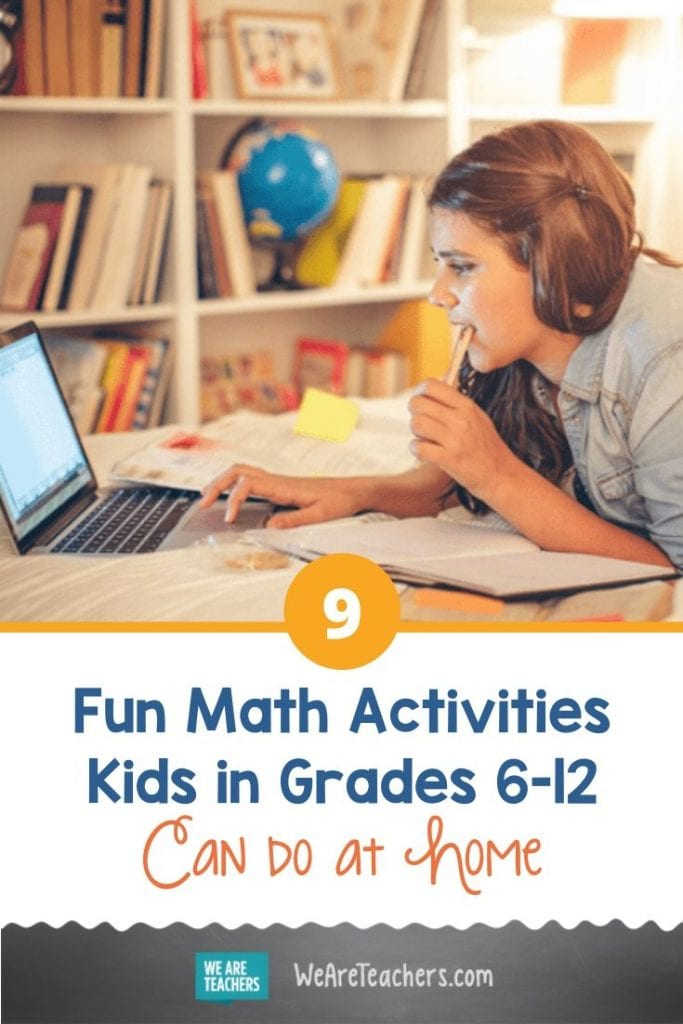What do triathlon training, comparison shopping, and painting-by-numbers have in common? They’re all fun math activities your students can do at home. How? LONG + LIVE + MATH At Home includes a free, curated collection of Carnegie Learning’s math resources for students in grades 6-12. The math lessons, skills practice resources, and educational videos help keep students motivated to learn math even when they’re not in the classroom.

Here are a few of our favorites:

## 1. Triathlon Training (Relating Distance, Rate, and Time, Grade 6)

Data is important in sports training. This lesson and video use graphs and ratios to determine average speeds and other information from data collected about a young athlete training for triathlons.

## 2. A Trip to the Moon (Representing Equivalent Ratios, Grade 6)

The ratio of weight on Earth: weight on the Moon is approximately 60 lb: 10 lb. In this activity, students use ratio tables to look at body weights on earth and determine the equivalent weights on the moon.

## 3. Math Football (Using Models to Understand Integer Addition, Grade 7)

A football game in math class? This activity is a great way to learn how to combine positive and negative numbers.

## 4. What is the Best Buy? (Introducing Unit Rates, Grade 7)

Do your students know how to figure out the best buy in a supermarket? This lesson on comparison shopping uses pictorial modeling to help understand unit rates.

## 5. It’s a Generational Thing (Properties of Powers with Integer Exponents, Grade 8)

A dog’s pedigree is traced as the generations of its ancestors are examined. Students use integer exponents as an efficient way to help express their findings in this fun activity.

## 6. DIY (Designing a Study and Analyzing the Results, Grades 9-12)

These DIY activities provide opportunities for real-world practical application of statistical analysis as students create their own study and analyze the results.

## 7. Get to the Point (Building Volume and Surface Area Formulas, Geometry)

This hands-on exploration helps students discover, develop, and understand formulas for volume and surface area of 3-D shapes.

## 8. Paint By Numbers (Art and Transformations, Algebra II)

Feeling creative? This is an open-ended exploration where transformations of functions and equations are used along with graph paper to create drawings.

## 9. MATHia (Individualized math instruction software, Grades 6-12)

Want to provide individualized math instruction for learning at home? You can get free access to MATHia, personalized software designed specifically for students to learn independently. Teachers can choose the content. Students work whenever they want, progressing through the learning path at their own pace. In addition, MATHia adapts to give students exactly what they need at any given moment.